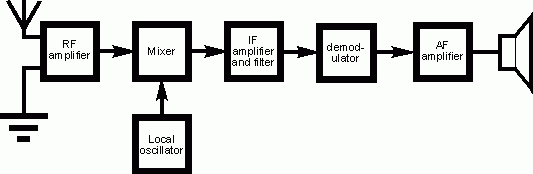# "Ham Radio Basics" Practice Test

Questions in test: 10
Time for Test: 10 minutes

1) The correct phonetic code for the word APPLE is:
2) The most commonly used Receiver design for amateur radio is :3) The ability of a radio receiver to separate signals that are close to each other in frequency is its:
4) What is a Multimeter?
5) What device is used to store electrical energy in an electrostatic field:
6) Radio waves travel at the speed of:
7) A current of 15 µA is equivalent to:
8) If a current of 2 amp flows through a 50 OHM resistor, what is the voltage across that resistor?
9) The word Attenuate means:
10) An electric current always consists of :